[ HOME ]   [ NEXT ]   [ BACK ]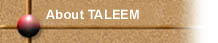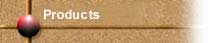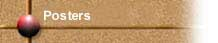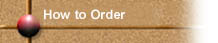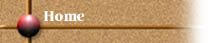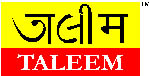A       D I V I S I O N      O F      E K L A V Y A      E D U C A T I O N      F O U N D A T I O N

 Code : TLM-M-008 Age : 8 - 10 yrs. Name: Fraction Flip Chart-1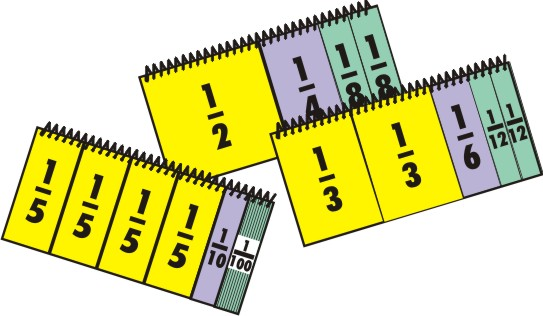Each set contains    Flip chart with 10 charts with each chart  having different fractions 1, 1/2, 1/4, 1/8,  1/3, 1/6, 1/12, 1/5, 1/10, 1/100.  This game is used  to demonstrate fraction relationships with unique set of laminated flip charts.

 How to use
 This game that we have here is called fraction flip chart. The first chart here is 1. This is one complete chart. Now we will divide this chart into two equal parts. So what we get is two parts of the same chart which are of equal size. So this is one half and this is another half. The denominator of this fraction 1/2 indicates the number of parts made of the original chart. Now we will divide this complete chart into 4 parts. So what we get is 4 equal parts of the same chart. This is one 1/2 th, this is another 1/4 th, this is third 1/4 th and this is the fourth 1/4 th part. You can see that the earlier 1/2 is actually formed of two equal parts of 1/4th each. So 1/4 is twice 1/4th or 1/4th is half of 1/2. The denominator of this fraction 1/4 indicates the number of parts made of the original chart. Now we will divide the chart further into eight equal parts. So what we have now is 8 equal parts each represented by 1/8. So this is one 1/8, this is second 1/8, this is third 1/8 and so onYou can see that the earlier 1/4 part is actually formed of two 1/8 parts. So 1/4th is twice 1/8th or 1/8th is half of 1/4th. In the similar fashion the second set of 1/3rd,  1/6th and 1/12th flip charts and the third set of 1/5th, 1/10th and 1/100th flip charts is to be explained to the children.

 Development aspects
 Any rational number can be represented in the form of a fraction.  Fraction is generally represented as a/b, where a and b are integers and b>0.  E.g. 1/2, 1/3, 1/4, 1/8, 1/10, 1/00 etc.

 Principle
 Children are introduced to the concept of fraction through graphical representation of fractions. It is done by associating different portions with their respective values. E.g. 1/4 is half of 1/2 and twice 1/8. A number 1/5 for instance can be expressed as twice 1/10 and 20 times 1/100. It is important that children link the parts with their respective values .

 Teaching Learning Materials (TLM) can make learning much more effective and fun in classrooms. Taleem is an initiative in this direction.

[ HOME ]   [ NEXT ]   [ BACK ]